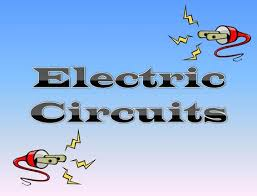Circuits and Electricity
3 years ago
kbartsc
Save
Edit
Host a game
Live GameLive
Homework
Solo Practice
Practice10 QuestionsShow answers
• Question 1
30 secondsQ. Label the circuit
closed circuit
complete circuit
incomplete circuit
working circuit
• Question 2
30 seconds
Q. A light bulb will shine brighter when
An extra light bulb is added to the circuit
A smaller battery is used
The switch is open in the circuit
• Question 3
30 seconds
Q. A complete circuit is an open circuit
True
False
• Question 4
30 secondsQ.
Circuit is open
Circuit is closed
circuit has a complete path for electricity to flow
This doesn't have to do with a circuit
• Question 5
30 secondsQ.
This is a series circuit, one path to flow
This is a parallel circuit, more than one path to flow
This is an incomplete circuit
This is a simple circuit
• Question 6
30 secondsQ.
Series circuit
Parallel circuit
open circuit
not a circuit
• Question 7
30 secondsQ. What would happen if the switch was opened?
nothing, the light bulbs would still work because there is a complete path
The light bulbs would not work because the path was broken
the battery would loose energy
the wires would be cooled from air
• Question 8
30 seconds
Q. All of the following are forms of energy you can create in a circuit EXCEPT
thermal and light
mechanical and sound
light and mechanical
sound and solar
• Question 9
30 secondsQ. The on switch shows
An open circuit where electricity can flow
A closed circuit that is also called complete
not related to circuits
An incomplete circuit that does not allow electricity to flow
• Question 10
30 secondsQ. This circuit